# USAGE OF OPERATIONAL AMPLIFIER AND ITS APPLICATION

``````                       **OPERATIONAL AMPLIFIER**
``````

An op-amp is a high gain electronic voltage amplifier which is DC coupled with differential input and usually single-ended output. Op-amps are widely used in analog circuits because of its versatility.an op-amp is capable of producing an output potential which is hundreds of thousands times larger than the potential difference between its input terminal. Op-amps had its beginning in analog computers. In which they were used to perform many mathematical operations in many linear, non-linear, and frequency dependent circuits. Op -amps are the most widely used electronic device today it is used in many industrial and scientific devices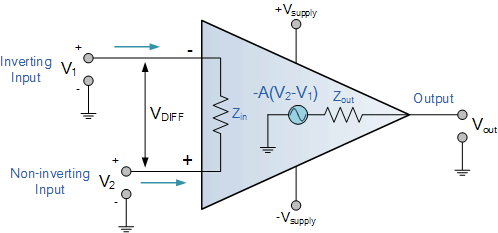Op-amps are basically called as building blocks of analog electronic circuits it is a linear device that has almost all the properties for Dc amplification. And therefore used mostly in signal conditioning, filtering or to perform mathematical operations like addition, subtraction, integration, and differentiation. An op-amp is mainly a voltage amplifying device which is to be used with external feedback components like resistors and capacitors between its input and output terminals. These feedback components determine the resulting function of the amplifier whether to be resistive or capacitive or both the amplifier can perform a variety of different operation hence it has the name operational amplifier

APPLICATIONS

AMPLIFICATION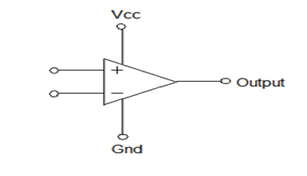An amplified output signal from the OpAmp is the difference between the two input signals.

if both the inputs are supplied with the same voltage the op-amp will take the difference between the two voltages and it will be 0. The op-amp will multiply this with its gain 1000,000 so the output is 0. When 2 volts is given to one input and 1 in other then op-amp will take its difference and multiply it with the gain that is 1 volt * 1000,000 but this gain is really high to reduce the gain feedback from output to input is done through a resistor.

INVERTING AMPLIFIER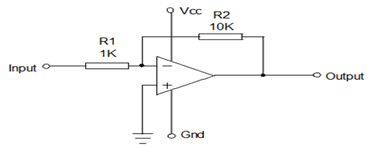This shows an inverting amplifier where the non-inverting input connected to the ground the resistors r1 and r2 are connected in such a way that r1 feeds the input signal while r2 returns the output to the inverting input here if the input is +ve output will be –ve and vice versa the voltage change at the output relative to input depends on the ratio of resistors

NON – INVERTING AMPLIFIER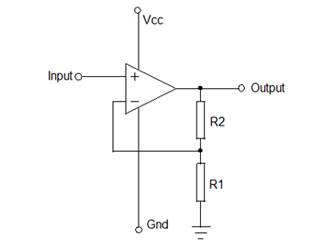Here the non-inverting input will receive the signal while inverting input is connected between r1 and r2. When the input signal moves either +ve or –ve the output will be in phase and keep the voltage at inverting input as that of the non-inverting input

COMPARATOR Op-amp compares the voltage applied in one input to the voltage applied in other any difference between the voltages even it is small it will drive the op-amp into saturation when the voltage applied to the inputs have same magnitude and polarity then op-amp o/p is 0

TYPES OF OPERATIONAL AMPLIFIER

VOLTAGE FOLLOWER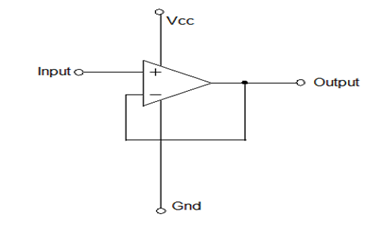It provides high input impedance low output impedance while the input voltage changes the output and the inverting input will change equally

SUMMING AMPLIFIER

This is a type of op-amp which can be used to sum signals to the input. the signal amplified by certain factors and made available at the output any number of the input signal can be summed by using an op-amp.INTEGRATOR Op-amp integrator is an operational amplifier that performs the mathematical operation of integration which means we cause the output to respond changes to the input voltage op-amp will produce an output voltage which is proportional to the integral of the input voltage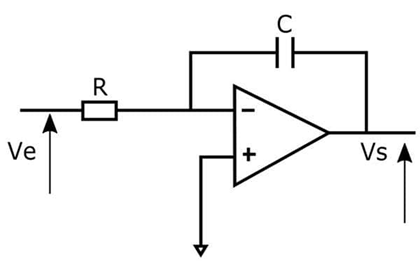By this we can use op-amp as an integrator a capacitor on the inverting feedback path and a resistor on the input inverting pin as shown in the figure if the input signal is very low frequency the capacitor acts like an open circuit and blocks the feedback voltage then the amplifier would be like a normal open-loop amplifier with high gain and the amplifier is saturated by the help of resistor parallel to the capacitor the circuit behaves like an inverting amplifier with a low frequency and the saturation is avoided.